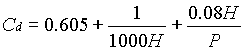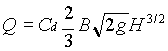### Flow Measurement - Rectangular Weir

A rectangular weir 0.75 m high and 1.5 m long is to be used for discharging water from a tank under a head of 0.5 m. Estimate the discharge (i) when it is used as a suppressed weir (ii) when it is used as a contract weir. Use Rehbock equation for estimating Cd in both cases.

Data:

Weir height (P) = 0.75 m

Width of weir (B) = 1.5 m

Formulae:

Rehbock equationH and P in meter

Suppressed weirContracted weirWhere n = number of contractions

Q = flow rate

Calculations:

1. Suppressed weir:
2. Cd = 0.605 + 1 / (1000 x 0.5) + 0.08 x 0.5 / 0.75 = 0.66

Q = 0.66 x (2/3) x 1.5 x (2 x 9.812)0.5 x 0.53/2 = 1.034 m3/sec

3. Contracted weir
4. Q = 0.66 x (2/3) x (1.5 - 0.1 x 2 x 0.5) x (2 x 9.812)0.5 x 0.53/2 = 0.965 m3/sec

HOME